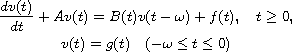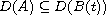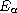Electron. J. Diff. Equ., Vol. 2014 (2014), No. 160, pp. 1-13.

### Stability of parabolic equations with unbounded operators acting on delay terms Allaberen Ashyralyev, Deniz Agirseven

Abstract:
In this article, we study the stability of the initial value problem for the delay differential equationin a Banach space E with the unbounded linear operators A and B(t) with dense domains. We establish stability estimates for the solution of this problem in fractional spaces. Also we obtain stability estimates in Holder norms for the solutions of the mixed problems for delay parabolic equations with Neumann condition with respect to space variables.

Submitted April 14, 2014. Published July 21, 2014.
Math Subject Classifications: 35K30.
Key Words: Delay parabolic equation; stability estimate; fractional space; Holder norm.

Show me the PDF file (240 KB), TEX file, and other files for this article.Allaberen Ashyralyev Department of Mathematics, Fatih University 34500 Buyukcekmece Istanbul, Turkey email: aashyr@fatih.edu.tr Deniz Agirseven Department of Mathematics, Trakya University 22030, Edirne, Turkey email: denizagirseven@yahoo.com